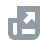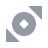Acheter des cryptos
Payer avec
Dérivés
Revenus passifs
Finance
NFT
Institution
Flux
USD

# FAQFonctionnalités du compteTutorielBinance Fan TokenBinance EarnDépôt/Retrait de CryptosAcheter des Cryptos (Fiat/P2P)Produits dérivés de cryptosFinanceAPISécuritéConditions d'utilisationBinance ConvertNFTVIP
Cet article n’est pas disponible actuellement dans votre langue. L’utilisation d’un outil de traduction automatique est recommandée pour l’anglais.
Accueil
Service client
FAQ
Produits dérivés de cryptos
Contrats à terme
Indice

2020-08-26 13:28
Index tracks and measures the performance of a basket of assets in a standardized way. There are two types of indices: single underlying price indices and composite price indices. The single underlying price indices mainly focus on tracking some specific underlying instrument price with the same underlying across different markets; while the composite price indices are combinations of different underlying instruments intended to measure the overall market (or subset) performance over time, the indices price usually calculated as weighted average price of the components.
The first index launched by Binance Futures is DEFI Composite Index. It tracks the market performance of a basket decentralized finance (DEFI) protocol tokens listed on Binance. It is calculated by using weighted averages of real time prices of the DEFI tokens on Binance and is denominated in USDT.
The constituents of DEFI Composite Index will be adjusted from time to time. Please refer to section 4.0 Rebalances for more detail.
2.0 Specifications of DEFI Composite Index
3.0 Index Calculation
Calculation example:
Step 1
To calculate Weighting Factor we need information from table below (The table updates once a week, please refer to the latest table in Section 5.0 “Constituents and Weighting Factor of DEFI Composite Index”）：
Weighting factor formula：
Take BAND as an example：
√Market Cap = √228138790 = 15104.263967502686
√Perpetual Volume = √462009026 = 21494.395222941257
(√Market Cap + √Perpetual Volume) / 1000
= (15104+21494) / 1000
=36598 / 1000
= 36.598

Use this method to calculate all 10 constitutes, add up to 484.947.
Then we get BAND weights in 36.598 / 484.947 = 7.55%
Calculate the weighting factor of each constitute in the index, we get Column F. (‘’factor’’: representing percentage of initial/adjusted value)
Step 2
* price of i coin at t time
For example at t time, index = 10000
Take BAND as an example:
Wit = 1000 * 7.55% / 12.61
= 5.98464
Price (initial/last rebalance) i.e. Column G，refers to the price index of each coin during rebalance, in other words, it is the price index used by USDT Futures Contracts).
We can see there are 5.98464 BAND in the Index.
Step 3
Divisor t, is the latest stable divisor, which ensures continuous change of index constituents/weight and the index price affected only by constituents price change. The divisor on base day is:：
Calculate the latest value (pi * wi), we get Column J.
Column J is also the latest price index (if the divisor remains unchanged and stays as its initial value 1000)
Take BAND as an example：
Latest value = pi * wi = 13.1998 * 5.98464= 78.996 USDT
Step 4
Calculate Index：
Because we have used the weighting factor in the current calculation, the Divisor does not change itself, and remains as the initial value 1000.
Therefore, Index t = the sum of Pi*Wit (*1000/1000) i.e. the sum of Column J in the table:
Index = 78.99625274 + 73.492 +69.297+ … +96.076 = 1007.821

4.0 Rebalances
4.1 Regular Rebalances
The indices constituents/weight will be rebalanced every week to measure the latest updated market.
When one or several constituents face drastic changes (e.g. delist or no organic volume), when special adjustment deemed necessary, there are two kinds of adjustment: exclude and recalculate weight (and Divisor t), exclude and fill up with constituents from the alternate list, recalculation also needed.
Here is an example to discuss how Divisor t ensures continuous change of index constituents/weight and the index price affected only by constituents price change.
We use table below as a reference for calculation:
Take LEND as an example,
Latest value (after rebalance) =1 *0.81= 0.81（E6*F6)
The latest value of all constitutes (after special rebalance) are summed up to 884.89 (i.e. sum of column H)
Latest divisor = Last divisor（1000）* Latest value (after rebalance) / Latest value (before rebalance)
= 1000 * sum of column H * sum of column I
=1000 * 884.89 / 995.8
=888.6
We have produced a new Divisor t, which is not equal to 1000.
Then we use below formula:
Index = Latest value (after rebalance) * 1000 / Latest divisor
=884.89 * 1000 / 888.6 = 995.8
We can see that the numbers in cell K3 and K5 are the same, which means that even if we rebalanced the weight of a constitute, we can use the Divisor to ensure the index price is stable and will be affected only by constituents' price change.